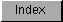```
The FFP transcendental math library contains entries for the
transcendental math functions sine, cosine, and square root. It resides on
disk and is opened by calling OpenLibrary() with "mathtrans.library" as
the argument.

#include <exec/types.h>
#include <libraries/mathffp.h>

#include <clib/mathffp_protos.h>
#include <clib/mathtrans_protos.h>

struct Library *MathTransBase;

VOID main()
{
if (MathTransBase = OpenLibrary("mathtrans.library",0))
{
.
.
.
CloseLibrary(MathTransBase);
}
else
printf("Can't open mathtrans.library\n");
}

The global variable MathTransBase is used internally for all future
library references.  Note that the transcendental math library is
dependent upon the basic math library, which it will open if it is not
open already.  If you want to use the basic math functions in conjunction
with the transcendental math functions however, you have to specifically
open the basic math library yourself.

FFP Transcendental Functions

```